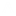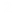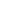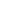```
1 {-
2
3 Pearl 3: Improving on Saddleback search
4
5 Definitions:
6 Given function f(x,y) -> z , where x,y,z are Natural numbers.
7 f( , ) is strictly increasing in both it's arguments.
8
9 Problem:
10 Write the function invert which returns all the pairs (x,y) such that
11 f(x,y)=z
12
13 Solution:
14
15 Two methods: One that 'kind off' goes along a 'line' from top-left to bottom right, walking on the 'iso-line'.
16 The second is based on binary search, with the right limits, on different rows/columns
17
18 -}
19 module P3 where
20
21 -- Test function
22 f (x,y) = x+2*y
23 --f (x,y) = 3*x+27*y+y^2
24 --f (x,y) = x^2+y^2+x+y
25
26
27 -- Brute force
28 -- Requires (z+1)^2 evaluations of f
29 invert1 f z = [(x,y) | x<-[0..z],y<-[0..z],f (x,y) == z]
30
32 -- Going from the top left to the bottom right, but moving to the right
33 -- in smart way, so NOT searching the whole triangle. More like searching
34 -- along a line.
35 invert2 f z = find2 (0,m) f z n
36                 where
37                     -- determine boundaries of 'box' to search"
38                     -- m on the y-axis, n on the x
39                     -- we will search in the (0,0) (m,n) box
40                     m = bsearch (\y->f(0,y)) (-1,z+1) z
41                     n = bsearch (\x->f(x,0)) (-1,z+1) z
42
43 find2 (u,v) f z n
44     | u > n || v < 0    =    []                            -- if we are out of the box: Stop
45     | z'<  z            =     find2 (u+1,v) f z n            -- we started from the TOP on the y-axis in every column
46                                                         -- so if we stepped down on the column and didn't find it,
47                                                         -- move one column to the right
48     | z'== z            =     (u,v) : find2 (u+1,v-1) f z n-- We found one!! go to the right
49     | z'>  z             =    find2 (u,v-1) f z n            -- Keep going down this column. we are still too large.
50     where
51         z' = f(u,v)
52
53
54
55 -- regular binary search
56 bsearch g (a,b) z
57     | a+1 == b         = a                 -- no more 'segment' left
58     | g m <= z        = bsearch g (m,b) z -- look at the top segment
59     | otherwise     = bsearch g (a,m) z -- look at the bottom segment
60     where
61         m = (a + b) `div` 2
62
63
64 -- Binary search-2D, full swing
65 invert3 f z =     find3 (0,m) (n,0) f z
66                 where
67                     m = bsearch (\y->f(0,y)) (-1,z+1) z
68                     n = bsearch (\x->f(x,0)) (-1,z+1) z
69
70 find3 (u,v) (r,s) f z
71     | u > r || v < s     = []    -- out of bounderies
72     | v-s <= r-u         = rfind (bsearch (\x->f(x,q)) (u-1,r+1) z)    -- Rows are longer than columns: search along row
73     | otherwise         = cfind (bsearch (\y->f(p,y)) (s-1,v+1) z)    -- Column search
74     where
75         p         = (u+r) `div` 2
76         q        = (v+s) `div` 2
77         rfind p = (if f (p,q) == z     then (p,q): find3 (u,v) (p-1,q+1) f z     -- Top-Left Rectangle
78                                     else find3 (u,v) (p,q+1) f z ) ++
79                     find3 (p+1,q-1) (r,s) f z                                -- Bottom-Right rectangle
80
81         cfind q = find3 (u,v) (p-1,q+1) f z ++                                -- Top-Left
82                   (if f (p,q) == z     then (p,q): find3 (p+1,q-1) (r,s) f z    -- Bottom-Right
83                                     else find3 (p+1,q) (r,s) f z )
84
85
86
87 -- Main
88
89 main = do
90     putStr "Brute force       : "
91     print \$ invert1 f 18
92     putStr "Saddleback search : "
93     print \$ invert2 f 18
94     putStr "Binary 2D search  : "
95     print \$ invert3 f 18
```